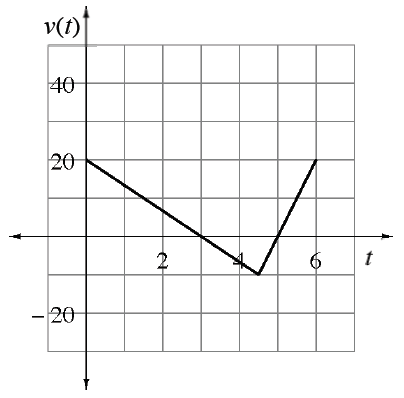### Home > APCALC > Chapter 4 > Lesson 4.3.1 > Problem4-111

4-111.

The graph at right shows the velocity (in miles per hour) of a car during a road trip. At time $t = 0$, the car was on the Golden Gate Bridge heading north.

1. Write a function for $v\left(t\right)$.

Write an equation for each line segment.

2. How far north has the car traveled at $t = 3$ hours? At $t = 4$ hours?

Since this is a velocity graph, calculate the area under the curve for the given interval.

$\int_0^3v(t)dt\text{ and }\int_0^4v(t)dt$

3. Explain what happened to the car for $3 ≤ t ≤ 5$ hours.

For $3 ≤ t ≤ 5$, the function values are negative. This is a velocity graph, so what does negative velocity tell you about position?

4. Set up an integral to represent the displacement from $0 ≤ t ≤ 6$.

$\text{displacement }=\int_{a}^{b}\text{velocity } dt$

5. Set up an integral to represent the total distance from $0 ≤ t ≤ 6$.

$\text{total distance }=\int_{a}^{b}\text{speed } dt=\int_{a}^{b}\left | \text{velocity} \right |dt$